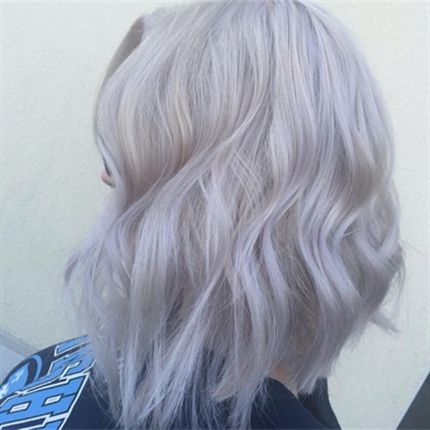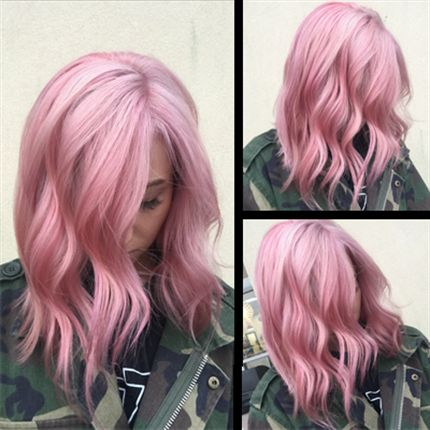Think Pink Color Formula
December 1, 2016

# Think Pink Color Formula• Formula A:

Paul Mitchell Violet Flash Toner + Equal parts 5-volume developer

• Formula B:

Redken Shades EQ 9v + Equal parts processing solution

• Formula C:

Redken Shades EQ 8v + Equal parts processing solution

• Formula D:

Pulp Riot 4 oz. Blush + ½ oz. Cupid

Winter goes hand-in-hand with dark and dreary days, which is exactly why we need fashion colors to add some life to the season! If your client is asking for a specific shade of pink, it can be hard to figure out which mix of colors will achieve the exact color she desires. But, if she’s asking for a true bubblegum pink, look no further than this “think pink” color formula from Kelly McCormick (@kellymccormickhair), a stylist at Salon 202 in Howell, N.J. Get the how-to!

Colorist: Kelly McCormick

## COLOR FORMULAS• Formula A:

Paul Mitchell Violet Flash Toner + Equal parts 5-volume developer

• Formula B:

Redken Shades EQ 9v + Equal parts processing solution

• Formula C:

Redken Shades EQ 8v + Equal parts processing solution

• Formula D:

Pulp Riot 4 oz. Blush + ½ oz. Cupid

## HOW-TO STEPS• 1

During the first session, lighten the roots with 30-volume developer and lightener. Process for 20 minutes, then run through at the sink.

•• Formula A:

Paul Mitchell Violet Flash Toner + Equal parts 5-volume developer

• Formula B:

Redken Shades EQ 9v + Equal parts processing solution

• Formula C:

Redken Shades EQ 8v + Equal parts processing solution

• Formula D:

Pulp Riot 4 oz. Blush + ½ oz. Cupid

2

Next, tone using Formula A and process for 5 minutes.

• 3

At the second session, perfect the blonde color by toning with Formula B for 3 minutes.

•• Formula A:

Paul Mitchell Violet Flash Toner + Equal parts 5-volume developer

• Formula B:

Redken Shades EQ 9v + Equal parts processing solution

• Formula C:

Redken Shades EQ 8v + Equal parts processing solution

• Formula D:

Pulp Riot 4 oz. Blush + ½ oz. Cupid

4

Lighten the roots with Formula C and process for 5 minutes. Then wash her hair with Shimmer Lights and towel-dry well.

• 5

To deepen the color, apply Formula D in small sections and process for 10 minutes.

•